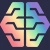PromptBaseExplore
ModelsMidjourneyDALL·EStable DiffusionGPTArt & IllustrationsAnimeCartoonPaintingIllustrationUnique StylesLogos & IconsLogoIconGraphics & DesignPatternProduct DesignProfile PictureProductivity & WritingProductivityWritingCodingMarketing & BusinessMarketingBusinessSocial MediaPhotographyPhotographyPhotography StyleGames & 3D3DFun & GamesVideo Game ArtGeneral
Home
Marketplace
Generate
Hire
Sell
🤖 GPT# Ultimate Math

TestedTipsNo reviews yet43

This prompt guides you through the fundamental nature of how you should ask ChatGPT a math related problems. Its use is not limited to the area of math and infinite. We'll guide you through to be able to learn from a simple prompt whenever you need it. You have a problem with your high school math? Here's a personal tutor just for you. You have problem learning university / college level maths? Here's the way through. You won't need any other math-related prompts after using this one.

\$3.99

Updated: 4 months ago

#### Prompt Details

Model
Chat - GPT-4 (gpt-4)
Token size
722 (\$0.0217 / call)
Example input
[mathematical term]: linearly independent, pre-image, vector, vertex, area, volume [topic]: calculus, pre-calculus, algebra, trigonometry [input]: x = 13, z = 82, N = ((1, 2), (3, 4))
Example output
We'll follow these steps: Write the matrices S and T: S = [[0, 1], [-1, 0]] T = [[0, -1], [-1, 0]] Multiply S and T to find their composition S o T: S o T = S * T = [[0, 1], [-1, 0]] * [[0, -1], [-1, 0]] = [[00 + 1(-1), 0*(-1) + 10], [-10 + 0*(-1), -1*(-1) + 0*0]] = [[-1, 0], [0, 1]] Choose a vector to transform, such as v = [3, 4]. Multiply the vector v by the matrix S o T: S o T * v = [[-1, 0], [0, 1]] * [3, 4] = [-13 + 04, 03 + 14] = [-3, 4] Therefore, the transformation S o T maps the vector [3, 4] to the vector [-3, 4]. To understand this result geometrically, you can visualize the effect of the individual transformations S and T on the vector [3, 4]: Applying S to [3, 4] rotates it 90 degrees counterclockwise and reflects it across the y-axis, resulting in the vector [-4, 3]. Applying T to [-4, 3] reflects it across the line y=-x, resulting in the vector [-3, 4]. So the composite transformation S o T has the same effect as first rotating the vector 90 degrees counterclockwise, then reflecting it across the y-axis, and finally reflecting it across the line y=-x, which results in the final vector [-3, 4]. *This prompt is not only limited to Calculus and teaches you how to use chatGPT properly to learn math.